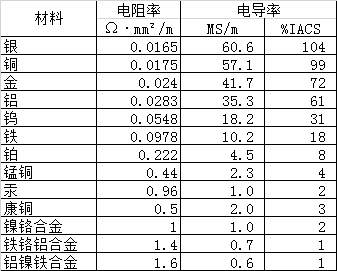R=ρ*L/S

ρ=R*S/L

σ=1/ρ

(%IACS) = σ(mS/m)/58.0*100%

(%IACS) = 1/(58.0*ρ(Ω·mm²/m))*100% = 0.017241/ρ(Ω·mm²/m)*100%

IACS的电导率单位就是%IACS，是一个百分数。表示为导电能力是国际退火铜标准的百分数。